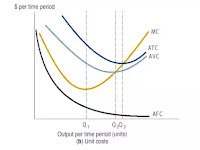# Mathematical Derivation of Cost Function

##Driving Short-run Cost Function from Cobb-Douglas Production Function

Normally, every firm face two problems while producing their product,

1.     How much quantity of goods to produce?

2.     And how much labor and capital is required to produce output most efficiently?

The production Function state relationship between output and input such as,

Q = ƒ (K, L)

Where,

Q is output or quantity

K is the input of capital

L is the input of Labor

Cobb-Douglas Production Function

Q = L^a K^b

Where,

Q is output

L is unit of labor

K is a unit of Capital

a & b = the parameter to be estimated.

Let a & b equally = 0.5

Q = L^0.5 K^0.5

Suppose cost components of a firm are as follow,

Price of a new machine – K = \$36 million

The wage of each worker – W = \$144 per day

Rental rate of capital or price of capital – r = \$200 per annum

“r” = (interest rate + Rate of Depreciation) x Price of capital

Lets determine the value of L

Q = L^0.5 36^0.5 = L^0.5 x 6

Therefore, L^0.5 = Q/6

L = (Q/6)^2 = Q^2/36

Total Cost (TC) = Variable Cost (VC) + Fixed Cost (FC)

TC = W*L + r*K

TC = 144*Q^2/36 + 200*36

TC = 4Q^2 + 7,200

VC = 4Q^2

FC = 7,200

Average total cost (ATC) = TC / Q = 4Q^2/Q + 7,200/Q

Therefore, ATC = 4Q + 7,200/Q

AVC = 4Q and AFC = 7,200/Q

We can calculate the Marginal Cost by taking first derivative of TC

TC = 4Q^2 + 7,200

⸫ MC = dTC/dQ = (4*2) Q^2-1 + 0 = 8Q

Minimum Cost condition where,

MC = ATC

8Q = 4Q + 7,200/Q

8Q – 4Q = 7,200/Q

4Q = 7,200/Q

Multiply both sides of equation by Q

Q(4Q) = (7,200/Q) Q

4Q2 =7,200

⸫ Q = (7,200/4)^1/2 = 42.43

Identical value MC and ATC obtained,

MC = 8 * 42.43 = 339.4

ATC= 4(42.43) + 7,200/42.43